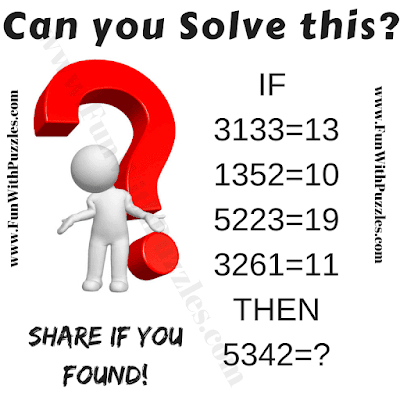This Clever Logical Riddle will test your logical reasoning skills. In this clever logical riddle, you are presented with some logical equations in the given puzzle picture. You need to decipher the logical reasoning behind these equation which makes these equations logically correct. Can you find the logical connection between the given numbers in this clever logical riddle and then solve for the missing number which will replace the question mark?Can you solve this clever logical riddle?

Answer of this "Clever Logical Riddle", is hidden as of now to avoid any spoiler. Do post your answers in the comments box. Also you can post your request to view the answer in the comment section. Please do give your best try to solve this brain teaser.

Unknown said...

17

Unknown said...

5342=?
5*2=10
3+4=7
10+7=17

Unknown said...

10